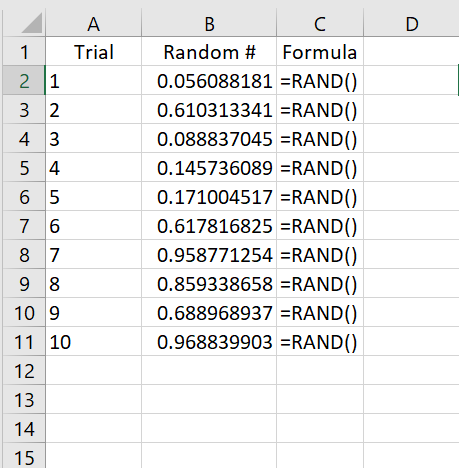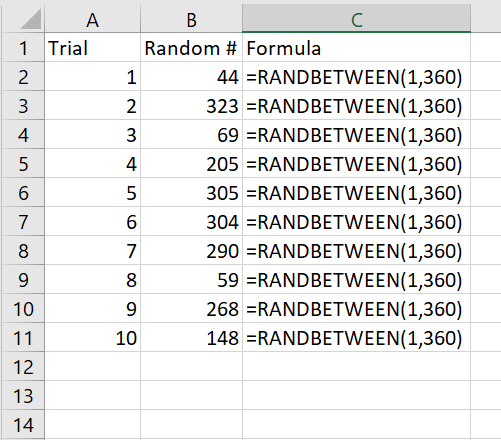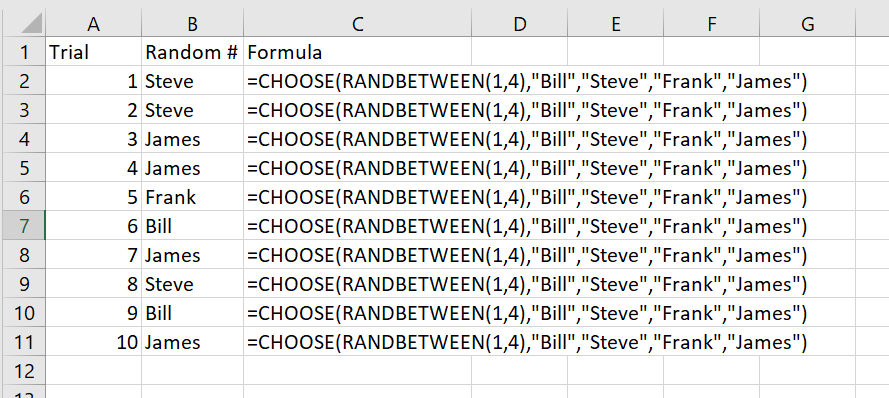# Excel Random Number Generator

There may be many cases where you need to create a random number generator. Luckily, Excel has a few native functions that will help achieve your goals. If you want to learn more about Excel,  Get 60% Off Online Excel Courses

## RAND()

When you type the RAND() function in a cell  you will get a number that is a random integer from 0 to 1. In theory, this means that approximately 50 percent of the time you will get a number that is less than 0.50 and the other 50 percent of the time you will get a number that is above 0.50. To get an idea of how this works lets take a look at the data below.

The RAND function takes no arguments to use it simply type =RAND()Once you have this function it will randomly generate a number,  simply press F9  key to generate a new random number. You can see from the image above that you only have to enter the formula to generate a random number from 0 to 1.

However, you might want to extend the range of your randomly generator number. You can do this by using another equation in Excel that deals with random numbers with a custom defined ranges. This is called RANDBETWEEN

## RANDBETWEEN()

The RANDBETWEEN(bottom, top) function is very similar to the RAND function. However, it takes a range which is set by a top and bottom number that is defined by the user. So, if you wanted to chose a random number between  1 and 360,  you would use the the following formula below

=RANDBETWEEN(1,360)## BONUS: RANDOM NAME GENERATOR

Create a random name generator in Excel with RANDBETWEEN Function.

So we have covered the random number generator. Now lets learn how to use what you have gained from the RANDBETWEEN function to generate names or any text by using the CHOOSE function. Using the CHOOSE function allows you to set an index and value associated with at index. So, for example 1 = Bill, 2 = Steve, and so on.  Combing these looks like the below.

=CHOOSE(RANDBETWEEN(1,4),”Bill”,”Steve”,”Frank”,”James”)